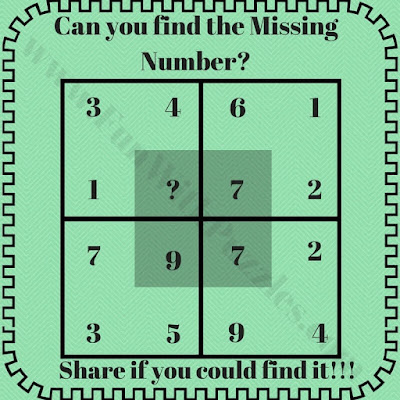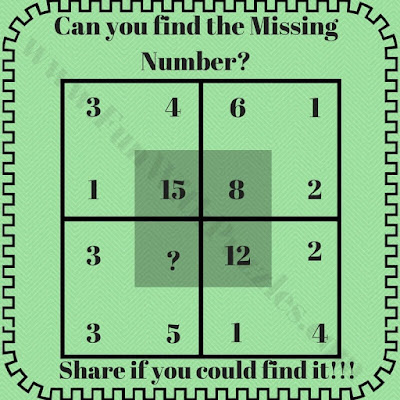## Tuesday, May 5, 2020

We keep on posting different types of puzzles on this website. Some puzzles are logical which will make you think logically. Some puzzles are observational which will help you to improve your visual skills. Some puzzles are mathematical which will not only help to sharpen your mathematical skill but also increase your interest in Mathematics. Today's puzzles are missing number puzzles which are mathematical. These are the extension of previous published similar puzzles with the title  "Awesome Missing Number Math Square Puzzles".
In these puzzles, a big square is divided into four smaller squares and each square is having four numbers which are related to each other with some mathematical pattern. One has to logical deduct this mathematical pattern and then find the value of the missing number.
So are you ready to find the missing number? Do post your answers with an explanation in the comment section.1. Can you find the missing numbers puzzle2. Easy math square question riddle3. Math puzzle to find the missing number4. Maths square photo puzzle question5. Mind breaking math square puzzle question

Answers and explanation of these missing number puzzles are given below. To understand the explanations of these maths missing number puzzles note below-mentioned notations.
Let us say D is the number in the shaded square.
Let A be number opposite to D and B, C be the other numbers which are opposite to each other
1. 19
The logic used in this missing number puzzle is as below
D = 2xA + B + C

2. 2
The logic used for this easy math puzzle is as below
D = B+C-A

3. 4
The logic used in this math puzzle is as below
A x D = B x C

4. 24
The logic used in the math photo puzzle question is as below
D = (B+C)xA

5. 9
Multiply the upper numbers to get the lower number with it is the unit's place written on the right side and ten's place digit is written on the left side.
e.g. 3x4 = 12, 6x7 = 42 etc.

1.1.Thanks for asking the explanation of answer to these missing number maths puzzles. Detailed explanation is added now for these puzzles. Please do post your comment in case something is still not clear regarding the answers and explanations of these puzzles.

2.What numbers will replace question mark for?
4 3 2 0 ?
8 6 2 8 ?

1.Give some more hints for these number pattern puzzles.

3.2+3=23,3+1=34,4+2=26,6+3=29,than 7+1=? Find that missing number

A comment doesn't cost a thing. Please drop a comment below to boost the author's morale.